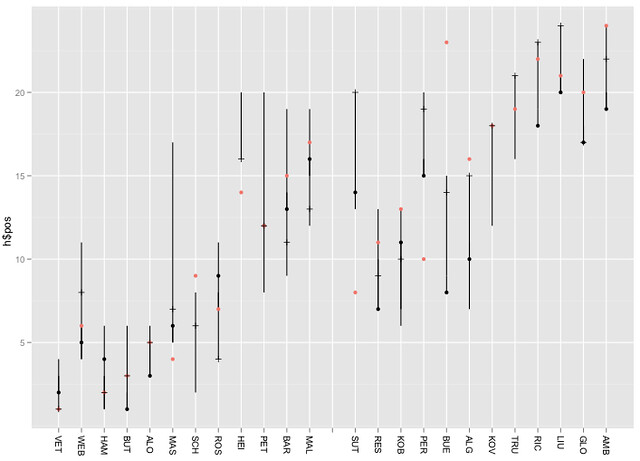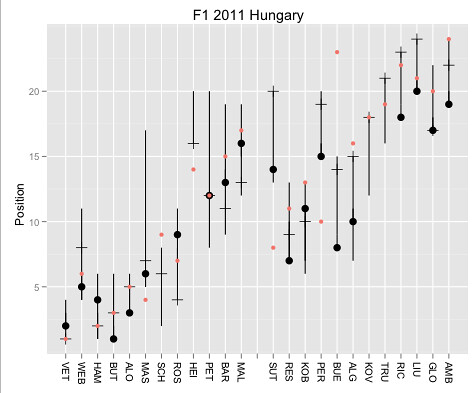Data Driven Story Discovery: Working Up a Multi-Layered Chart

How many different dimensions (or “columns” in a dataset where each row represents a different sample and each column a different measurement taken as part of that sample) can you plot on a chart?

Two are obvious: X and Y values, which are ideal for representing continuous numerical variables. If you’re plotting points, as in a scatterplot, the size and the colour of the point allow you to represent two further dimensions. Using different symbols to plot the points gives you another dimension. So we’re up to five, at least.

Whilst I was playing with ggplot over the weekend, I fell into this view over F1 timing data:It shows the range of positions held by each car over the course of the race (cars are identified by car number on the x-axis, 1 to 25 (there is no 13), and range of positions on the y-axis. The colour, uninterestingly, relates to car number.

If you follow any of the F1 blogs, you’ll quite often see references to “driver of the day” discussions (e.g. Who Was Your Driver of the Day). Which got me wondering…could a variant of the above chart provide a summary of the race as a whole that would, at a glance, suggest which drivers had “interesting” races?

For example, if a driver took on a wide range of race positions during the race, might this mean they had an exciting time of it? If every car retained the same couple of positions throughout the race, was it a procession?

Having generated the base chart using the ggplot web interface, I grabbed the code used to generate it and took it into RStudio.

What was lacking in the original view was an explicit statement about the position of each car at the end of the race. But we can add this using a point, if we know the final race position*:ggplot() + geom_step(aes(x=h\$car, y=h\$pos, group=h\$car)) + scale_x_discrete(limits = c('VET','WEB','HAM','BUT','ALO','MAS','SCH','ROS','HEI','PET','BAR','MAL','','SUT','RES','KOB','PER','BUE','ALG','KOV','TRU','RIC','LIU','GLO','AMB')) + xlab(NULL) + opts(axis.text.x=theme_text(angle=-90, hjust=0)) + geom_point(aes(x=k\$driverNum, y=k\$classification))

Let’s add it using a different coloured mark:+geom_point(aes(x=k\$driverNum, y=k\$grid,col='red'))

(Note that in the above example, the points are layered in the order they appear in the command line, with lower layers first. So if a car finishes (black dot) in the position it started (red dot), we will only see the red dot. If we make the lower layer dot slightly larger, we would then get a target effect in this case.)

The chart as we now have it shows where a driver started, where they finished and how many different race positions they found themselves in. So for example, we see BUE rapidly improved on his grid position at the start of the race and made progress through the race, but SUT went backwards from the off, as did PER.

Hmm… lots of race action seems to have happened during the first lap, but we’re not getting much sense of it… Maybe we need to add in the position of the car at the end of the first lap too. Unfortunately, the data I was using did not contain the actual grid/starting position (it just contains the positions at the end of each lap), but we can pull this data in from elsewhere… Using another dot to represent this piece of data might get confusing, so let’s use a line (set the symbol type using pch=3):+geom_point(aes(x=l\$car, y=l\$pos, pch=3))

Now we have a chart that shows the range of positions occupied by each car, their grid position, final race position and position at the end of the first lap Using different symbol types and colours we can distinguish between them. (For a presentation graphic, we also need a legend…) By using different sized symbols and layers, we could also display multiple dimensions at the same x, y co-ordinate.library("ggplot2")
h=hun_2011proximity
//in racestats, convert DNF etc to NA
k=hun_2011racestatsx
l=subset(h,lap==1)
ggplot() + geom_step(aes(x=h\$car, y=h\$pos, group=h\$car)) + scale_x_discrete(limits =c('VET','WEB','HAM','BUT','ALO','MAS','SCH','ROS','HEI','PET','BAR','MAL','','SUT','RES','KOB','PER','BUE','ALG','KOV','TRU','RIC','LIU','GLO','AMB')) + xlab(NULL) + opts(title="F1 2011 Hungary", axis.text.x=theme_text(angle=-90, hjust=0)) + geom_point(aes(x=l\$car, y=l\$pos, pch=3, size=2)) + geom_point(aes(x=k\$driverNum, y=k\$classification,size=2), label='Final') + geom_point(aes(x=k\$driverNum, y=k\$grid, col='red')) + ylab("Position")

A title can be added to the post using:

I still haven’t worked out how to do a caption that will display:
– [black dot] “Final Race Position”
– [red dot] “Grid Position”
– [tick mark] “End of lap 1”

Any ideas?

The y-axis gridline on the half is also misleading. To add gridlines for each position, add in +scale_y_discrete(breaks=1:24) or to label them as well +scale_y_discrete(breaks=1:24,limits=1:24)So what do we learn from this? Layers can be handy and allow us to construct charts with different overlaid data sets. The order of the layers can make things easier or harder to read. Different symbol types work differently well with each other when overlaid. The same symbol shape in different sizes and appropriate layer ordering allows you to overlay points and still see them data. Symbol styles give the chart a grammar (I used circles for the start and end of the race positions, for example, and a line for the first lap position).

Lots of little things, in other words. But lots of little things that can allow us to add more to a chart and still keep it legible (arguably! I don’t claim to be a graphic designer, I’m just trying to find ways of communicating different dimensions within a data set).

* it strikes me that actually we could plot the final race position under the final classification. (On occasion, the race stewards penalise drivers so the classified result is different to the positions the cars ended the race in. Maybe we should make that eventuality evident?)

PS as @sidepodcast pointed out, I really should remove non-existent driver 13. I guess this means converting x-axis to a categorical one?

Author: Tony Hirst

I'm a Senior Lecturer at The Open University, with an interest in #opendata policy and practice, as well as general web tinkering...